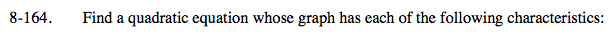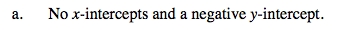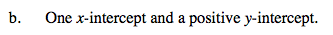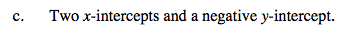Home > A2C > Chapter 8 > Lesson 8.2.4 > Problem8-164

8-164.
1. Find a quadratic equation whose graph has each of the following characteristics: 8-164 HW eTool (Desmos). Homework Help ✎

1. No x-intercepts and a negative y-intercept.

2. One x-intercept and a positive y-intercept.

3. Two x-intercepts and a negative y-intercept.Write the equation of an inverted parabola with a vertex below the x-axis.This parabola will sit on the x-axis.Shift the graph of y = x² down.

Use the eTool below to explore the problem.
Click the link at right for the full version of the eTool: A2C 8-164 HW eTool